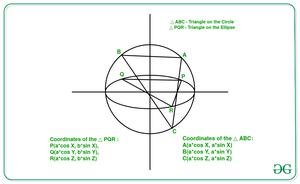# Calculate ratio of area of a triangle inscribed in an Ellipse and the triangle formed by corresponding points on auxiliary circle

Given two integers A and B representing the lengths of semi-major and semi-minor axes of an ellipse, the task is to calculate the ratio of any triangle inscribed in the ellipse and that of the triangle formed by the corresponding points on its auxiliary circle.

Examples:

Input: A = 1, B = 2
Output: 2
Explanation: Ratio = B / A = 2 / 1 = 2

Input: A = 2, B = 3
Output: 1.5

Approach:The idea is based on the following mathematical formula:

• Let the 3 points on the ellipse be P(a cosX, b sinX), Q(a cosY, b sinY), R(a cosZ, b sinZ).
• Therefore, the corresponding points on the auxiliary circles are A(a cosX, a sinX), B(a cosY, a sinY), C(a cosZ, a sinZ).
• Now, using the formula to calculate the area of triangle using the given points of the triangle.

Area(PQR) / Area(ABC) = b / a

Follow the steps below to solve the problem:

Below is the implementation of the above approach:

## C++

 `// C++ program for the above approach` `#include ` `using` `namespace` `std;`   `// Function to calculate ratio of a` `// triangle inscribed in an ellipse to` `// the triangle on the auxiliary circle` `void` `triangleArea(``int` `a, ``int` `b)` `{` `    ``// Stores the ratio of the` `    ``// semi-major to semi-minor axes` `    ``double` `ratio = (``double``)b / a;`   `    ``// Print the ratio` `    ``cout << ratio;` `}`   `// Driver Code` `int` `main()` `{` `    ``int` `a = 1, b = 2;` `    ``triangleArea(a, b);`   `    ``return` `0;` `}`

## Java

 `// Java program for the above approach` `class` `GFG{` ` `  `// Function to calculate ratio of a` `// triangle inscribed in an ellipse to` `// the triangle on the auxiliary circle` `static` `void` `triangleArea(``int` `a, ``int` `b)` `{` `    `  `    ``// Stores the ratio of the` `    ``// semi-major to semi-minor axes` `    ``double` `ratio = (``double``)b / a;`   `    ``// Print the ratio` `    ``System.out.println(ratio);` `}`   `// Driver Code` `public` `static` `void` `main(String args[])` `{` `    ``int` `a = ``1``, b = ``2``;` `    `  `    ``triangleArea(a, b);` `}` `}`   `// This code is contributed by AnkThon`

## Python3

 `# Python3 program for the above approach`   `# Function to calculate ratio of a` `# triangle inscribed in an ellipse to` `# the triangle on the auxiliary circle` `def` `triangleArea(a, b):`   `    ``# Stores the ratio of the` `    ``# semi-major to semi-minor axes` `    ``ratio ``=` `b ``/` `a`   `    ``# Print the ratio` `    ``print``(ratio)`   `# Driver Code` `if` `__name__ ``=``=` `"__main__"` `:`   `    ``a ``=` `1` `    ``b ``=` `2` `    `  `    ``triangleArea(a, b)`   `# This code is contributed by AnkThon`

## C#

 `// C# program for the above approach` `using` `System;` `using` `System.Collections.Generic;`   `class` `GFG{` ` `  `// Function to calculate ratio of a` `// triangle inscribed in an ellipse to` `// the triangle on the auxiliary circle` `static` `void` `triangleArea(``int` `a, ``int` `b)` `{` `    `  `    ``// Stores the ratio of the` `    ``// semi-major to semi-minor axes` `    ``double` `ratio = (``double``)b / a;`   `    ``// Print the ratio` `    ``Console.WriteLine(ratio);` `}`   `// Driver Code` `public` `static` `void` `Main()` `{` `    ``int` `a = 1, b = 2;` `    `  `    ``triangleArea(a, b);` `}` `}`   `// This code is contributed by bgangwar59`

## Javascript

 ``

Output:

`2`

Time Complexity: O(1)
Auxiliary Space: O(1)

Feeling lost in the world of random DSA topics, wasting time without progress? It's time for a change! Join our DSA course, where we'll guide you on an exciting journey to master DSA efficiently and on schedule.
Ready to dive in? Explore our Free Demo Content and join our DSA course, trusted by over 100,000 geeks!

Previous
Next